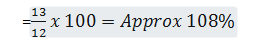New Students Offer - Use Code HELLO

# IBPS PO / CLERK Pre Exam 2016 – Quantitative Aptitude Quiz

Directions (Q.1-Q.5) : Study the following information carefully and answer the given questions below .1. What is the total population of village V1, V2 and V5 together ?
1) 191809
2) 191617
3) 197308
4) 191607
5) None of these

2. If the male population of village V8 is 48735, then what will be the female population of that village ?
1) 24960
2) 24860
3) 24780
4) None of these
5) 25980

3. What will be the difference in the population of village V3, V6 and V8 together and that of the village V1, V2 and V4 together?
1) None of these
2) 9896
3) 9736
4) 9086
5) 9826

4. The population of village V7 is what per cent of the population of village V1 ?
1) 104%
2) 106%
3) 108%
4) None of these
5) 105%

5. Which village has population more than 75000 ?
1) V4
2) V2
3) V3
4) V1
5) None of these

Directions(Q.6-Q.10): Study the following bar graph carefully and answer the questions given below :
The graph shows the number of persons using different modes of transport over the years.

6. What is the difference between the total number of persons travelling by Airlines in 2012, 2014 and 2015 and that travelling by Rail from 2011 and 2013 ?
1) 280
2) 300
3) 480
4) 320
5) None of these

7. In 2013 , the number of persons travelling by Bus, is approximately what percent of the number of persons travelling by Bus, Rail and Airlines together in that year?
1) 47%
2) 45%
3) 42%
4) 43%
5) None of these

8. What is the total number of persons travelling by Airlines in all the years together ?
1) 2060
2) 2150
3) 2080
4) 2050
5) None of these

9. What is the ratio of the number of persons travelling by Airlines in 2012 and 2013 together to the number of persons travelling by Bus in 2015 ?
1) 4 : 3
2) 5 : 4
3) 3 : 2
4) 5 : 3
5) None of these

10. What is the average number of persons travelling by Bus in all the years together ?
1) 620
2) 750
3) None of these
4) 680
5) 640

### Answer Key & Solution :

=2. 1) Female population of V8

= 15x 4913 – 48735 = 24960

3. 5) Required difference

= [(14+10+15) –(12+16+9)] x 4913 = 9826

4. 3) Required Percentage

=5. 2) Required percent be more thanOnly V2 has more than 15% population.

6. 2) Required difference

= (400+550+300) – (500+450) = 300

7. 1) Required percent

== 450+400+350+550+300 = 2050

9. 3) Required ratio

= 750 : 500

= 3:2

10. 5) Required average